# Pandas数据处理-map、apply、applymap详解

``````boolean=[True,False]
gender=["男","女"]
color=["white","black","yellow"]
data=pd.DataFrame({
"height":np.random.randint(150,190,100),
"weight":np.random.randint(40,90,100),
"smoker":[boolean[x] for x in np.random.randint(0,2,100)],
"gender":[gender[x] for x in np.random.randint(0,2,100)],
"age":np.random.randint(15,90,100),
"color":[color[x] for x in np.random.randint(0,len(color),100) ]
}
)
``````

# Series数据处理

``````#①使用字典进行映射
data["gender"] = data["gender"].map({"男":1, "女":0})
#②使用函数
def gender_map(x):
gender = 1 if x == "男" else 0
return gender
#注意这里传入的是函数名，不带括号
data["gender"] = data["gender"].map(gender_map)``````

# apply

``````def apply_age(x,bias):
return x+bias

#以元组的方式传入额外的参数
data["age"] = data["age"].apply(apply_age,args=(-3,))``````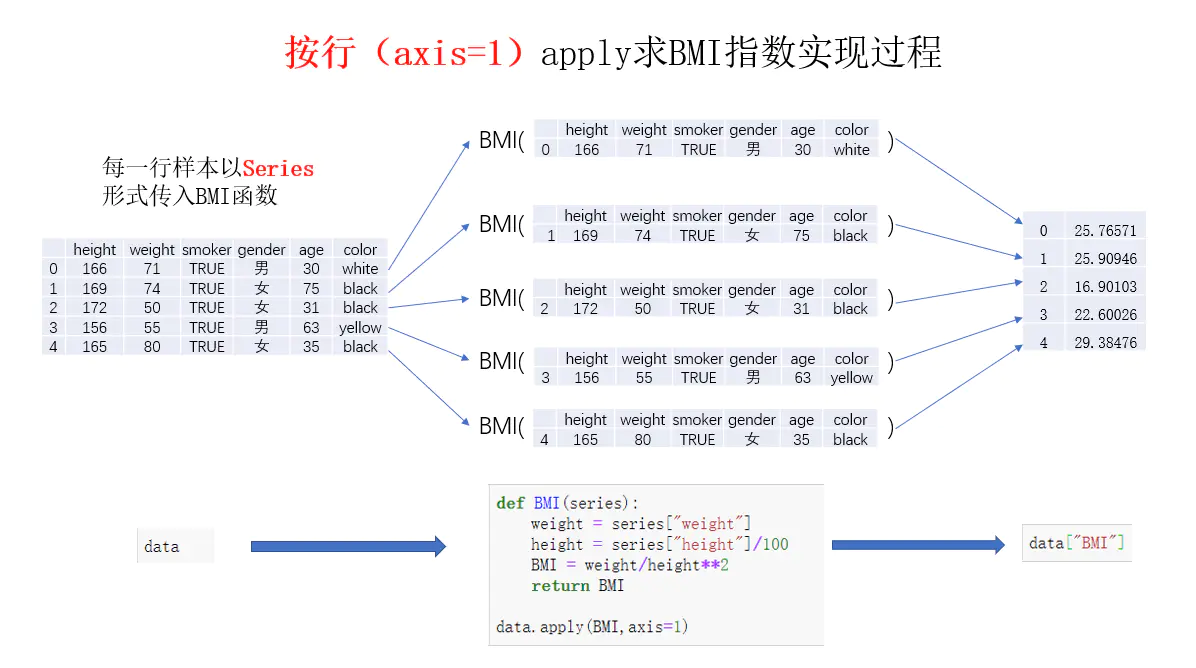# DataFrame数据处理

## apply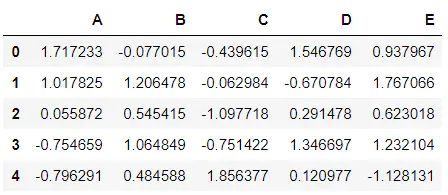``````# 沿着0轴求和
data[["height","weight","age"]].apply(np.sum, axis=0)

# 沿着0轴取对数
data[["height","weight","age"]].apply(np.log, axis=0)``````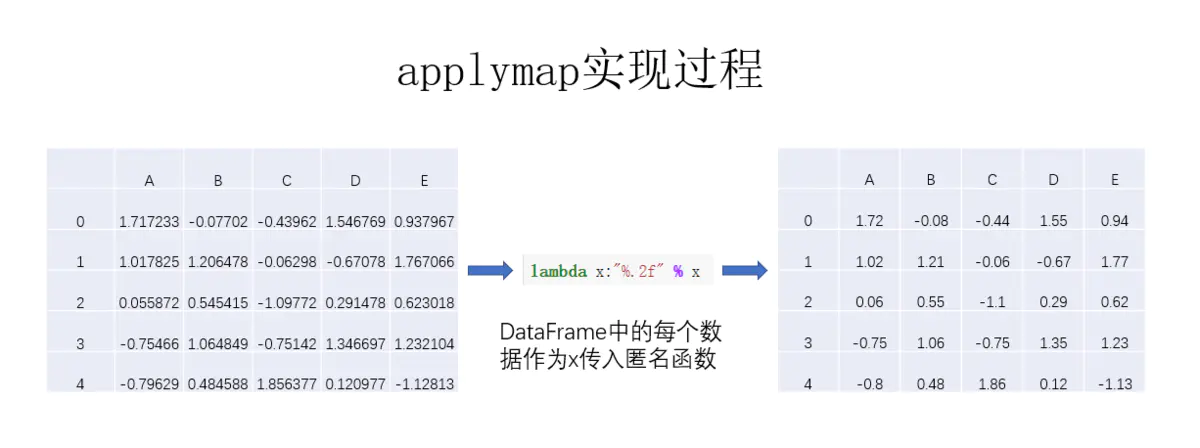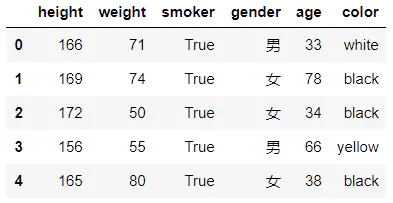``````def BMI(series):
weight = series["weight"]
height = series["height"]/100
BMI = weight/height**2
return BMI

data["BMI"] = data.apply(BMI,axis=1)``````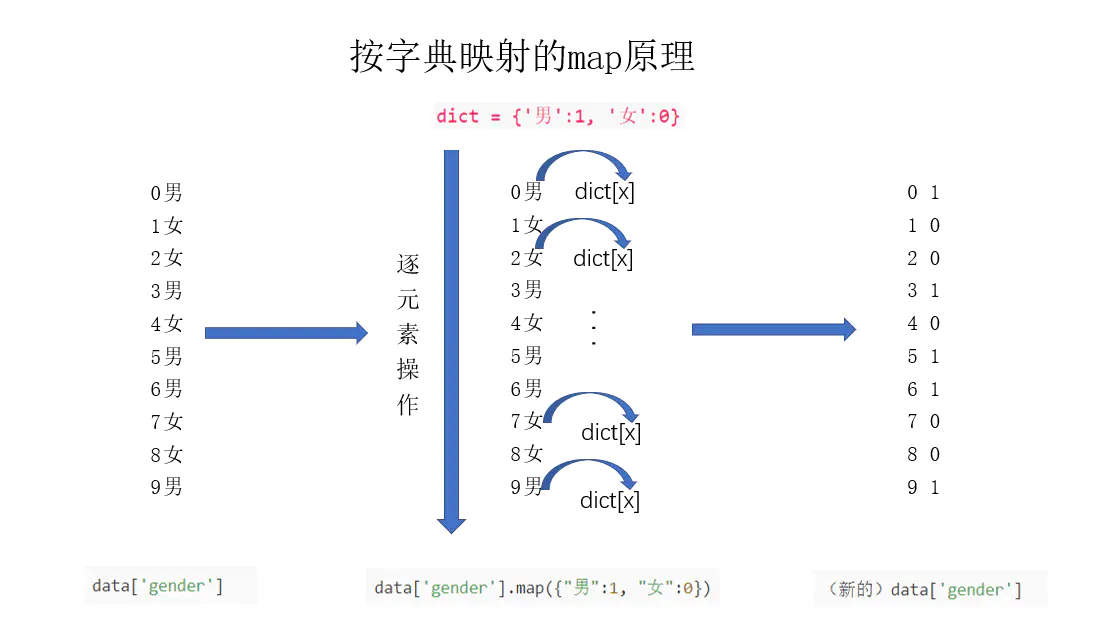1. 当 axis=0 时，对 每列columns 执行指定函数；当 axis=1 时，对 每行row 执行指定函数。 2. 无论 axis=0 还是 axis=1 ，其传入指定函数的默认形式均为 Series ，可以通过设置 raw=True 传入 numpy数组 。 3. 对每个Series执行结果后，会将结果整合在一起返回（若想有返回值，定义函数时需要 return 相应的值） 4. 当然， DataFrame 的 apply 和 Series 的 apply 一样，也能接收更复杂的函数，如传入参数等，实现 原理是一样的，具体用法详见官方文档。

# applymap

applymap 的用法比较简单，会对 DataFrame 中的每个单元格执行指定函数的操作，虽然用途不如 apply 广泛，但在某些场合下还是比较有用的，如下面这个例子。

``````df = pd.DataFrame(
{
"A":np.random.randn(5),
"B":np.random.randn(5),
"C":np.random.randn(5),
"D":np.random.randn(5),
"E":np.random.randn(5),     } )``````
``df.applymap(lambda x:"%.2f" % x)``

https://www.jianshu.com/p/e76861ed1815